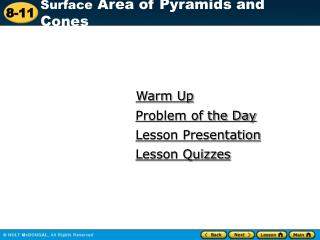Download PresentationWarm Up

# Warm Up - PowerPoint PPT PresentationDownload Presentation## Warm Up

- - - - - - - - - - - - - - - - - - - - - - - - - - - E N D - - - - - - - - - - - - - - - - - - - - - - - - - - -
##### Presentation Transcript

1. Warm Up Problem of the Day Lesson Presentation Lesson Quizzes

2. Warm Up Find the perimeter or circumference of each figure. Use 3.14 for . 1. 2. 30 cm 138.16 ft

3. Problem of the Day In a survey, 69 people were asked whether they prefer hamburgers or hot dogs. Thirty-three people said they prefer hot dogs. What percent of the people surveyed said they prefer hamburgers? about 52%

4. Sunshine State Standards MA.7.G.2.1 Justify and apply formulas for surface area..of…pyramids…and cones. Also MA.7.G.2.2

5. Vocabulary regular pyramid slant height of a pyramid slant height of a cone

6. The base of a regular pyramid is a regular polygon, and the faces are congruent isosceles triangles. The diagram shows a square pyramid. The blue dashed line labeled l is the slant height of the pyramid, the distance from the vertex to the midpoint of an edge of the base.

7. 1 2 S = lw + Pl 1 2 S = (9 • 9) + (36)(10)‏ Additional Example 1A: Finding the Surface Area of a Pyramid Find the surface area of the pyramid. 1 2 S = B + Pl Use the formula. B = lw Substitute. P = 4(9) = 36 S = 81 + 180 Add. S = 261 m2 The surface area is 261 square meters.

8. 1 2 1 2 S = bh + Pl 1 2 1 2 S = (7)(6) + (21)(8)‏ Additional Example 1B: Finding the Surface Area of a Pyramid Find the surface area of the pyramid. 1 2 S = B + Pl Use the formula. B= ½bh. Substitute. P = 3(7) = 21 S = 21 + 84 Add. S = 105 in2 The surface area is 105 square inches.

9. 5 m 1 2 S = lw + Pl 3 m 3 m 1 2 S = (3 • 3) + (12)(5)‏ Check It Out: Example 1A Find the surface area of the pyramid. 1 2 S = B + Pl Use the formula. B= lw. Substitute. P = 4(3) = 12 S = 9 + 30 Add. S = 39 m2 The surface area is 39 square meters.

10. 4 in. 1 2 1 2 S = bh + Pl 1 2 1 2 S = (4)(3) + (12)(6)‏ 6 in. 4 in. 4 in. 3 in. Check It Out: Example 1B Find the surface area of the pyramid 1 2 S = B + Pl Use the formula. B= ½bh. Substitute. P = 3(4) = 12 S = 6 + 36 Add. S = 42 in2 The surface area is 42 square inches.

11. The diagram shows a cone and its net. The blue dashed line is the slant height of the cone, the distance from the vertex to a point on the edge of the base.

12. Additional Example 2: Finding the Surface Area of a Cone Find the surface area of the cone. Use 3.14 for . S = r2 + rl Use the formula. S ≈ (3.14)(32) + (3.14)(3)(10)‏ Substitute. S ≈ 28.26 + 94.2 Multiply. S ≈ 122.46 Add. The surface area is about 122.46 square centimeters.

13. Check It Out: Example 2 Find the surface area of the cone. Use 3.14 for . S = r2 + rl Use the formula. S ≈ (3.14)(42) + (3.14)(4)(10)‏ Substitute. S ≈ 50.24 + 125.6 Multiply. S ≈ 175.84 Add. The surface area is about 175.84 square centimeters.

14. Lesson Quizzes Standard Lesson Quiz Lesson Quiz for Student Response Systems

15. Lesson Quiz 1. Find the surface area of the pyramid. 2. Find the surface area of the cone. Use 3.14 for . 236.25 m2 219.8 ft2 3. A house being built is to have a roof in the shape of a square pyramid. The sides of the square base are 21 feet long and the slant height will be 12 feet. What will the surface area of the roof be? (Hint: The base of the pyramid is not part of the roof.)‏ 504 ft²

16. Lesson Quiz for Student Response Systems 1. Find the surface area of a regular pyramid with a base of 12 feet by 12 feet and a slant height of 14 feet. A. 2016 ft2 B. 816ft2 C. 480ft2 D. 240 ft2

17. Lesson Quiz for Student Response Systems 2. Find the surface area of a cone with a radius of 3 feet and a slant height of 6 feet. Use 3.14 for . A. 18ft2 B. 56.52 ft2 C. 75.36ft2 D. 84.78ft2